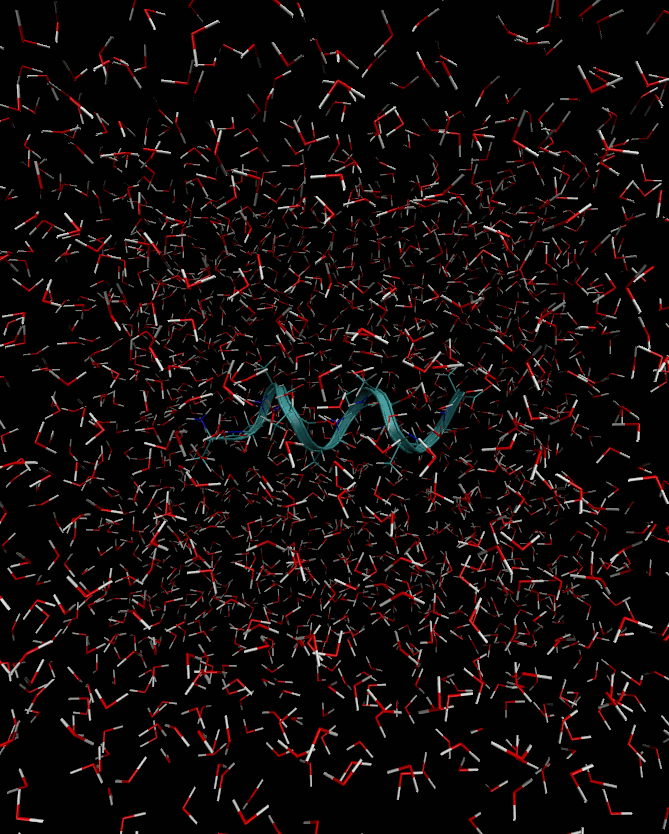#CP2K Open Source Molecular Dynamics

### Site Tools

exercises:2017_uzh_acpc2:prot_fol

# Protein Folding in Solution

In this exercise, you will calculate the protein folding free energy in aqueous solution using thermodynamic integration, a method based on molecular dynamics (MD). The protein will be described by the empirical force field, CHARMM, http://mackerell.umaryland.edu/charmm_ff.shtml

## Background

A model protein you will have to deal with is the alanine decapeptide. The folding/unfolding will be achieved by stretching/compressing the chain and fixing the distance between the end carbon atoms in it: atoms 7 and 98. This distance is called a collective variable. At each distance one runs the MD simulation (constrained MD) to extract the time-averaged forces acting on the collective variable, $F(x)$. Then, a free energy difference can be calculated via thermodynamic integration (TI):

\begin{equation} \Delta A = -\int_a^b F(x)\, dx \end{equation}

Here $a$ and $b$ are the initial and the final values of the collective variable. TI is a general method, which can be applied to a variety of processes, e.g. phase transitions, electron transfer etc.

deca_ala.pdb (protein data base) file contains the coordinates

deca_ala.psf (protein structure file) file contains connectivity data

par_all27_prot_lipid.inp contains the force field parameters

md_1836.inp is the CP2K input file

Open the deca_ala.pdb protein data bank format file with vmd. Create a new representation for the protein, e.g. of type Ribbon to observe the alpha-helix.## Task 2: Perform constrained MD simulations

For that you have to run MD for different values of the distance between atoms 7 and 98, in each run it will be constrained. In the original file md_1836.inp it is set to $18.36$ Å as is in the deca_ala.pdb file.

1. Run CP2K with md_1836.inp
2. Copy md_1836.inp to smth. like md_1536.inp;
3. Modify the PROJECT_NAME and TARGET value in the CONSTRAINT section for a new value: here 15.36;
4. Run CP2K with the new input file;
5. Repeat for several values in the range $15$ to $20$ Å.
• To avoid confusion, try to perfrom every task in a new directory
• You may increase or decrease the number of MD steps, which is set to 5000 in the file, to speed-up the calculation or else get a better statiscics.

### Constraint section TO BE modified for constrained MD

constraint section
 &CONSTRAINT
&COLLECTIVE
COLVAR 1
INTERMOLECULAR
TARGET [angstrom] 18.36
&END COLLECTIVE
&LAGRANGE_MULTIPLIERS
COMMON_ITERATION_LEVELS 1
&END
&END CONSTRAINT

## Task 3: Evaluate the free energy difference

⇒ Each constrained MD will produce a .LagrangeMultLog-files, which look like this:

Shake  Lagrangian Multipliers:           -63.547262596
Rattle Lagrangian Multipliers:            63.240598387
Shake  Lagrangian Multipliers:            -0.326901815
Rattle Lagrangian Multipliers:            -0.318145579
Make sure that you get the units right. The Largange multipliers are written in atomic units (Hartree/bohr), while the distances are in Angstrom.
• From these files you can calculate the average Lagrange multiplier of the Shake-algorithm like this:
grep Shake yourprojectname.LagrangeMultLog | awk '{c++ ; s=s+$4}END{print s/c}' • The average Lagrange multiplier is the average force$F(x)$required to constrain the atoms at the distance$x$. • From these forces the free energy difference can be obtained via TI (see Background) • Calculate$\Delta A\$ numerically using the trapezoidal rule (or equivalent) with EXCEL, ORIGIN or any scripting language.